# Introduction

There is not much reason to write another package for making simple ordination plots with ggplot. Gavin Simpson wrote his ggvegan package to provide autoplot methods for several classes of vegan ordination objects with ggplot. Phyloseq has been available for several years now, and includes wrappers for making ordinations and plotting them. The plot functions usually work with ordination objects made with vegan functions, too. The ggord package makes bi- and tri-plots with ordinations made with vegan and other packages. And it is really not difficult to write your own code - examples and scripts abound on the web. But I am not aware of a package including functions for implementing several vegan style plots I frequently use. Fitting environmental variables as vectors to an ordination with envfit, for example. Or delineating treatment groups with hulls, spiders, and ellipses with vegan’s family of ordi- functions. Hence my foray into the field with this package, ggordiplots.

# Hulls, Spiders & Ellipses with gg_ordiplot

vegan includes a family of functions for delineating treatment groups in an ordination plot: ordihull for enclosing all points for a treatment within a polygon, ordispider for linking all points for a treatment to their group centroid with line segments, and ordiellipse for scaling ellipses about group centroids in a variety of ways. I have collected all of these features into a single function, gg_ordiplot; each feature may be turned on or off with a logical as an argument. Usage (copied from the documentation available by entering ?gg_ordiplot) is:

gg_ordiplot(ord, groups, scaling = 1, choices = c(1, 2), kind = c("sd", "se", "ehull"),
conf = NULL, show.groups = "all", ellipse = TRUE, label = FALSE, hull = FALSE,
spiders = FALSE, plot = TRUE)

And the simple example given in the documentation is:

suppressPackageStartupMessages(library(vegan))
suppressPackageStartupMessages(library(ggplot2))
suppressPackageStartupMessages(library(ggordiplots))
data("dune")
data("dune.env")
dune.hel <- decostand(dune, method = "hellinger")
ord <- rda(dune.hel)
gg_ordiplot(ord, groups = dune.env$Management, pt.size = 3)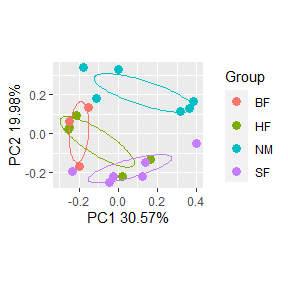By default, ellipses indicating one standard deviation about the centroid are plotted for each group (i.e. kind = “sd”). This can be changed to give the standard errors about the group centroids: gg_ordiplot(ord, groups = dune.env$Management, pt.size = 3, kind = "se")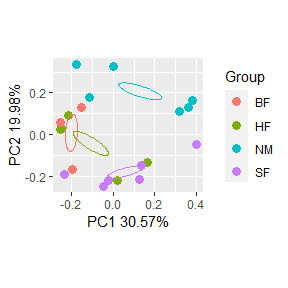If kind equals “se”, or “sd”, confidence intervals may be shown by setting conf to a numeric value. For example, show 95% confidence intervals for the standard errors:

gg_ordiplot(ord, groups = dune.env$Management, kind = "se", conf = 0.95, pt.size = 3)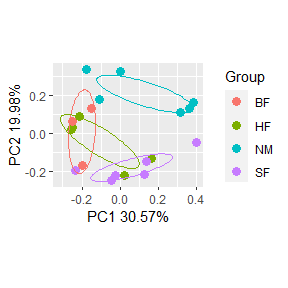Axes plotted may be chosen with choices of axes and ellipses (or other features) limited to a subset of the treatment groups with show.groups. For example, plot ordination axes 2 and 3, and draw ellipses for only BF and HF: gg_ordiplot(ord, groups = dune.env$Management, choices = c(2, 3), show.groups = c("BF",
"HF"), kind = "se", pt.size = 3, conf = 0.95)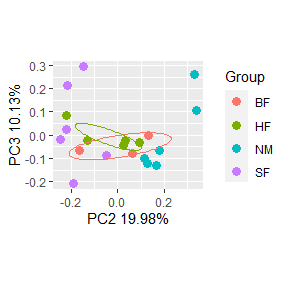As a final example, indicate treatment groups with hulls, labels and spiders instead of ellipses.

gg_ordiplot(ord, groups = dune.env$Management, hull = TRUE, label = TRUE, spiders = TRUE, ellipse = FALSE, pt.size = 3, plot = TRUE)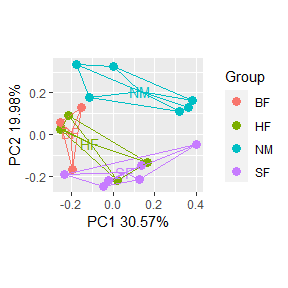# Displaying envfit Results Three functions in this package have to do with displaying relationships between site ordinations (based on species presences or abundances, i.e. community composition) and environmental variables. vegan’s envfit function makes linear fits between the variables and the ordination axes. These can then be displayed as vectors originating at the center of the plot. Projections of sites on these vectors should order the sites with respect to the variable. But the fit may not be linear. For individual variables, linearity can be checked graphically by making contour (or surface) plots, and by making bubble plots. In the first case, contours of the value of the variable are fit to the ordination plot. In the second, the site symbols are sized in proportion to the value of the variable. ## gg_envfit Usage for this function is: gg_envfit(ord, env, groups = NA, scaling = 1, choices = c(1, 2), perm = 999, alpha = 0.05, angle = 20, len = 0.5, unit = "cm", arrow.col = "red", pt.size = 3, plot = TRUE) And a simple example copied from the documentation is: data(varespec) data(varechem) vare.dist <- vegdist(varespec, method = "bray") set.seed(123) vare.mds <- monoMDS(vare.dist) set.seed(123) gg_envfit(ord = vare.mds, env = varechem, perm = 9999, pt.size = 2, alpha = 0.2)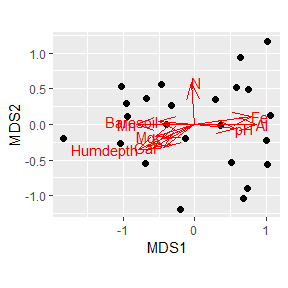As with gg_ordiplot, the function silently returns a list of the data frames (df_ord and df_arrows) used for the plot and the plot itself. They can be captured, examined and used to make modified plots as explained in the other vignette (“Modifying ggordiplots Plots”) included in this package. ## gg_ordisurf and gg_ordibubble The print method for envfit gives a table including the r2 and p.value (Pr>r) for each variable. set.seed(123) envfit(vare.mds, env = varechem, permutations = 9999) ## ## ***VECTORS ## ## MDS1 MDS2 r2 Pr(>r) ## N -0.08438 0.99643 0.2244 0.0628 . ## P -0.79760 -0.60318 0.1893 0.1128 ## K -0.91829 -0.39591 0.1549 0.1674 ## Ca -0.87347 -0.48688 0.2836 0.0287 * ## Mg -0.95770 -0.28777 0.2134 0.0810 . ## S -0.46045 -0.88769 0.0502 0.5873 ## Al 1.00000 0.00057 0.4819 0.0012 ** ## Fe 0.99076 0.13563 0.4072 0.0033 ** ## Mn -1.00000 0.00224 0.4183 0.0026 ** ## Zn -0.99761 -0.06912 0.1391 0.2076 ## Mo 0.77127 0.63651 0.0482 0.5980 ## Baresoil -0.99749 0.07085 0.1640 0.1564 ## Humdepth -0.91408 -0.40554 0.4758 0.0019 ** ## pH 0.99413 -0.10823 0.2062 0.0926 . ## --- ## Signif. codes: 0 '***' 0.001 '**' 0.01 '*' 0.05 '.' 0.1 ' ' 1 ## Permutation: free ## Number of permutations: 9999 I used a fairly large value of alpha in the gg_envfit plot above so that vectors for variables with weak correlations would still be included in the plot. And of course they all plotted as vectors, i.e. straight lines, because that is the way the model works. Let’s compare variables with strong and weak correlations using contour and bubble plots. For gg_ordisurf you may have to try different values of binwidth to get a plot that you like. The default value is calculated as the difference between the minimum and maximum values of the variable divided by 15. Larger values give fewer contours and vice versa. gg_ordisurf(ord = vare.mds, env.var = varechem$Al, binwidth = 20, pt.size = 1, var.label = "Aluminum")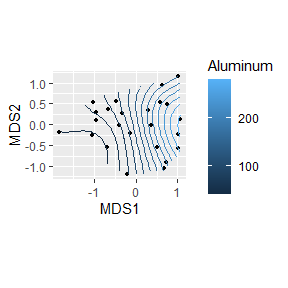gg_ordibubble(ord = vare.mds, env.var = varechem$Al, var.label = "Aluminum")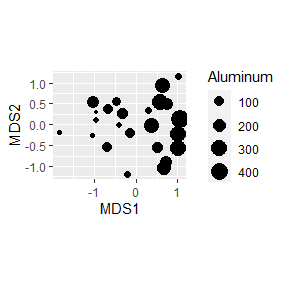The contours are close to parallel and both plots show that the Al concentration increases from left to right, in the same direction as the vector in the previous plot. Here we can compare directions because we are using the same ordination data for all of the plots. That is, we are not re-running the ordination each time. If we were, we would have to be more careful in making comparisons with directions. The signs along the axes have no real meaning, and axes can flip unexpectedly. This is especially true of NMDS which is essentially a randomization process. This is why I set the randomization seed, because otherwise the axes could flip when I compiled the document and would not match the text I had written. But axes can flip with other methods, too, if different algorithms are used or samples are added or removed. Now make the same types of plots for a variable with a weak correlation, % of ground that is bare soil. gg_ordisurf(ord = vare.mds, env.var = varechem$Baresoil, binwidth = 5, var.label = "Bare Soil")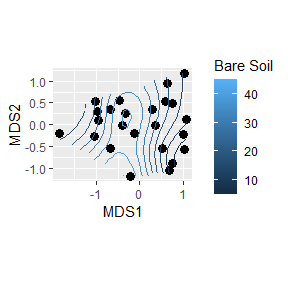gg_ordibubble(ord = vare.mds, env.var = varechem$Baresoil, var.label = "Bare Soil")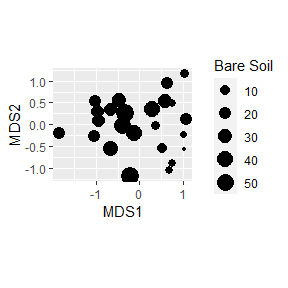This relationship is obviously not linear. The value peaks near the center of the first axis (i.e.is monotonic) and there is no clear relationship with the second axis. I tried making plots for molybdenum (Mo), but the correlation was so bad that the function to fit contours failed. From the bubble plot we can see why. gg_ordibubble(ord = vare.mds, env.var = varechem$Mo, var.label = "Molybdenum")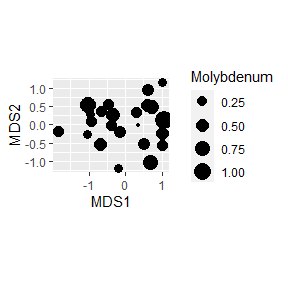gg_ordisurf and gg_ordibubble also silently return lists of the data frames used and the plots made. You may examine, use, and modify these with the methods you have learned above.

# gg_ordicluster

ordicluster is another member of vegan’s family of ordi- functions. It overlays a cluster diagram on an ordination plot. This is something I rarely use, but I have included the function gg_ordicluster to make the same plots with ggplot2. It accepts the same arguments as ordicluster (except display cannot be changed from “sites”), plus I have added functionality for showing treatment groups by mapping them to symbols (shapes in ggplot2). Usage is :

gg_ordicluster(ord, cluster, treatments = NA, choices = c(1, 2), prune = 0, col = 1,
plot = TRUE)

ord is an ordination object, and cl is the result from hclust based on the same distance matrix as the ordination. Kindt & Coe (BiodiverisityR) recommend single linkage clustering be used to evaluate how well ordination reflects clustering. The simple example from the documentation is:

data("dune")
dune.bray <- vegdist(dune, method = "bray")
ord <- cmdscale(dune.bray, k = nrow(dune) - 1, eig = TRUE, add = TRUE)
cl <- hclust(dune.bray, method = "single")
gg_ordicluster(ord, cluster = cl)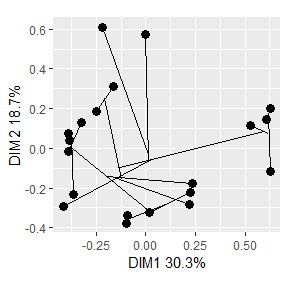And to demonstrate results with additional arguments:

data(dune.env)
cl <- hclust(dune.bray, method = "complete")
gg_ordicluster(ord, treatments = dune.env\$Management, cluster = cl, prune = 3, col = cutree(cl,
4), pt.size = 2)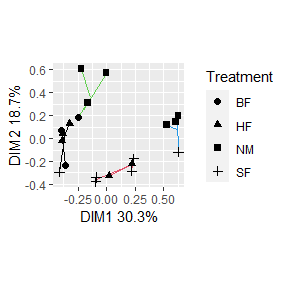# Modifying Plots

See the other vignette in this package, “Modifying ggordiplots Plots,” for information on how all of the above plots may be customized.